# Triangle perimeter

Calculate the triangle perimeter whose sides are in ratio 3: 5: 7 and the longest side is 17.5 cm long.

Result

o =  37.5 cm

#### Solution:Try calculation via our triangle calculator.

Leave us a comment of example and its solution (i.e. if it is still somewhat unclear...):

Showing 0 comments:Be the first to comment!#### To solve this example are needed these knowledge from mathematics:

See also our trigonometric triangle calculator.

## Next similar examples:

1. Arm-legCalculate the length of the base of an isosceles triangle with a circumference 224 cm if the arm length is 68 cm.
2. Sides of triangle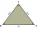Triangle circumference with two identical sides is 117cm. The third side measures 44cm. How many cms do you measure one of the same sides?
3. Isosceles triangleThe circumference of the isosceles triangle is 32.5 dm. Base length is 153 cm. How long is the leg of this triangle?
4. MedianThe median of the triangle LMN is away from vertex N 84 cm. Calculate the length of the median, which start at N.
5. Tree shadowThe shadow of the tree is 16 meters long. Shadow of two meters high tourist sign beside standing is 3.2 meters long. What height has tree (in meters)?
6. AnglesIn the triangle ABC, the ratio of angles is: a:b = 4: 5. The angle c is 36°. How big are the angles a, b?
7. Acute angles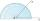Sizes of acute angles in the right-angled triangle are in the ratio 1: 3. What is size of the larger of them?
8. Angles ratioThe internal angles of a triangle are in ratio 1:4:5 What kind of triangle is it? (solve internal angles and write down and discuss)
9. Center traverseIt is true that the middle traverse bisects the triangle?
10. TriangleProve whether you can construct a triangle ABC, if a=9 cm, b=10 cm, c=4 cm.
11. Table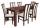The circumference of the rectangle table is 420 cm. Length to width ratio is 5:2 . Calculate table dimensions and dimensions reduced in the ratio 3:5
12. ParallelogramCalculate the missing side of a parallelogram, if you know the perimeter o and one side: a) o = 7.2 cm; b = 1.8 cm b) o = 5.4 cm; a = 1.9 cm
13. Guppies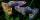Audrey has some guppies in a fish tank. The ration of the oranges guppies to silver guppies is 3:5. She has 12y oranges guppies. Write the number of silver guppies she has in terms of y
14. Map 3Map scale is M = 1: 25000 . Two cottages which are shown on the map are actually 15 km away. What is its distance on the map?
15. Cows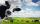4 cows spent 16 bags of hay in 5 days. How many bags of hay sacks is needed for 5 cows for seven days?
16. Train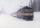The train passes part of the line for 95 minutes at speed 75 km/h. What speed would have to go in order to shorten the driving time of 20 minutes?
17. Holidays - on poolChildren's tickets to the swimming pool stands x € for an adult is € 2 more expensive. There was m children in the swimming pool and adults three times less. How many euros make treasurer for pool entry?